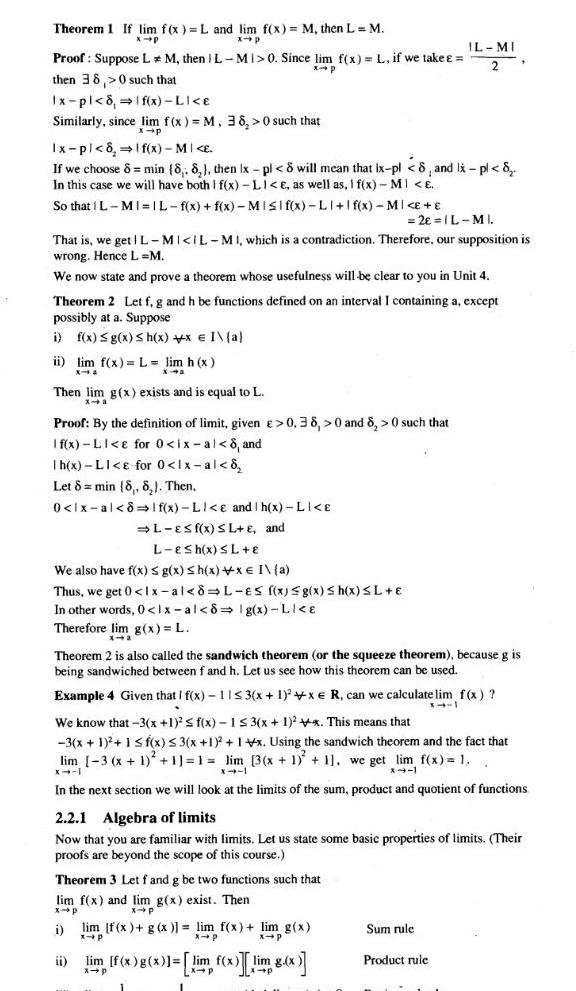Question:

# Theorem 1 If lim f x L and lim f x M then L M x p x p Proof

Last updated: 9/17/2023Theorem 1 If lim f x L and lim f x M then L M x p x p Proof Suppose LM then IL MI 0 Since lim f x L if we take x p then 38 0 such that Ix pl 8 1 f x LI e Similarly since lim f x M 38 0 such that x p Ix pl 8 If x MI E If we choose 8 min 8 8 then Ix pl 8 will mean that ix pl 8 and Ix pl 8 In this case we will have both I f x LI e as well as I f x MI E So that IL MI IL f x f x MISIf x LI f x MI E 2e L MI That is we get L MI IL MI which is a contradiction Therefore our supposition is wrong Hence L M We now state and prove a theorem whose usefulness will be clear to you in Unit 4 Theorem 2 Let f g and h be functions defined on an interval I containing a except possibly at a Suppose i f x g x h x x I a ii lim f x L lim h x X Then lim g x exists and is equal to L x a Proof By the definition of limit given 0 38 0 and 8 0 such that If x LI e for 0 1x al 8 and h x LI for 0 lx al 8 Let 8 min 8 8 Then 0 lx al 8 1 f x LI e and h x LI E L ES f x SL E and L esh x L E We also have f x g x h x x I a Thus we get 0 lx al 8 L es f x g x h x SL E In other words 0 lx al 8 Ig x LI E Therefore lim g x L Theorem 2 is also called the sandwich theorem or the squeeze theorem because g is being sandwiched between f and h Let us see how this theorem can be used Example 4 Given that I f x 11 3 x 1 x e R can we calculate lim f x X 11 IL MI 2 We know that 3 x 1 f x 1 3 x 1 2x This means that 3 x 1 1 f x 3 x 1 1 x Using the sandwich theorem and the fact that lim 3 x 1 1 1 lim 3 x 1 1 we get lim f x 1 X 4 1 x 1 x4 1 In the next section we will look at the limits of the sum product and quotient of functions Theorem 3 Let f and g be two functions such that lim f x and lim g x exist Then x p x p i 2 2 1 Algebra of limits Now that you are familiar with limits Let us state some basic properties of limits Their proofs are beyond the scope of this course ii lim f x g x lim f x lim g x x p lim f x g x lim f x im 8 x g x x p Sum rule Product rule